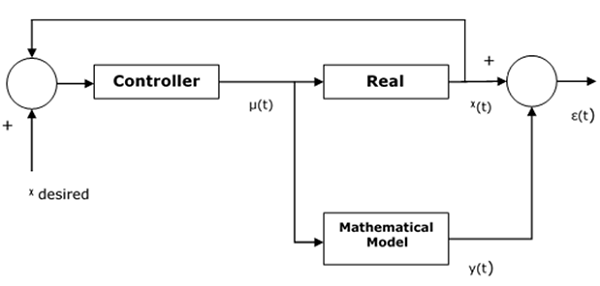In this chapter, we will discuss what is an Adaptive Fuzzy Controller and how it works. Adaptive Fuzzy Controller is designed with some adjustable parameters along with an embedded mechanism for adjusting them. Adaptive controller has been used for improving the performance of controller.

## Basic Steps for Implementing Adaptive Algorithm

Let us now discuss the basic steps for implementing adaptive algorithm.

• Collection of observable data − The observable data is collected to calculate the performance of controller.

• Adjustment of controller parameters − Now with the help of controller performance, calculation of adjustment of controller parameters would be done.

• Improvement in performance of controller − In this step, the controller parameters are adjusted to improve the performance of controller.

## Operational Concepts

Design of a controller is based on an assumed mathematical model that resembles a real system. The error between actual system and its mathematical representation is calculated and if it is relatively insignificant than the model is assumed to work effectively.

A threshold constant that sets a boundary for the effectiveness of a controller, also exists. The control input is fed into both the real system and mathematical model. Here, assume $x\left ( t \right )$ is the output of the real system and $y\left ( t \right )$ is the output of the mathematical model. Then the error $\epsilon \left ( t \right )$ can be calculated as follows −

$$\epsilon \left ( t \right ) = x\left ( t \right ) - y\left ( t \right )$$

Here, $x$ desired is the output we want from the system and $\mu \left ( t \right )$ is the output coming from controller and going to both real as well as mathematical model.

The following diagram shows how the error function is tracked between output of a real system and Mathematical model −## Parameterization of System

A fuzzy controller the design of which is based on the fuzzy mathematical model will have the following form of fuzzy rules −

Rule 1 − IF $x_1\left ( t_n \right )\in X_{11} \: AND...AND\: x_i\left ( t_n \right )\in X_{1i}$

THEN $\mu _1\left ( t_n \right ) = K_{11}x_1\left ( t_n \right ) + K_{12}x_2\left ( t_n \right ) \: +...+ \: K_{1i}x_i\left ( t_n \right )$

Rule 2 − IF $x_1\left ( t_n \right )\in X_{21} \: AND...AND \: x_i\left ( t_n \right )\in X_{2i}$

THEN $\mu _2\left ( t_n \right ) = K_{21}x_1\left ( t_n \right ) + K_{22}x_2\left ( t_n \right ) \: +...+ \: K_{2i}x_i\left ( t_n \right )$

.

.

.

Rule j − IF $x_1\left ( t_n \right )\in X_{k1} \: AND...AND \: x_i\left ( t_n \right )\in X_{ki}$

THEN $\mu _j\left ( t_n \right ) = K_{j1}x_1\left ( t_n \right ) + K_{j2}x_2\left ( t_n \right ) \: +...+ \: K_{ji}x_i\left ( t_n \right )$

The above set of parameters characterizes the controller.

The controller parameters are adjusted to improve the performance of controller. The process of calculating the adjustment to the parameters is the adjusting mechanism.

Mathematically, let $\theta ^\left ( n \right )$ be a set of parameters to be adjusted at time $t = t_n$. The adjustment can be the recalculation of the parameters,

$$\theta ^\left ( n \right ) = \Theta \left ( D_0,\: D_1, \: ..., \:D_n \right )$$

Here $D_n$ is the data collected at time $t = t_n$.

Now this formulation is reformulated by the update of the parameter set based on its previous value as,

$$\theta ^\left ( n \right ) = \phi ( \theta ^{n-1}, \: D_n)$$

## Parameters for selecting an Adaptive Fuzzy Controller

The following parameters need to be considered for selecting an adaptive fuzzy controller −

• Can the system be approximated entirely by a fuzzy model?

• If a system can be approximated entirely by a fuzzy model, are the parameters of this fuzzy model readily available or must they be determined online?

• If a system cannot be approximated entirely by a fuzzy model, can it be approximated piecewise by a set of fuzzy model?

• If a system can be approximated by a set of fuzzy models, are these models having the same format with different parameters or are they having different formats?

• If a system can be approximated by a set of fuzzy models having the same format, each with a different set of parameters, are these parameter sets readily available or must they be determined online?